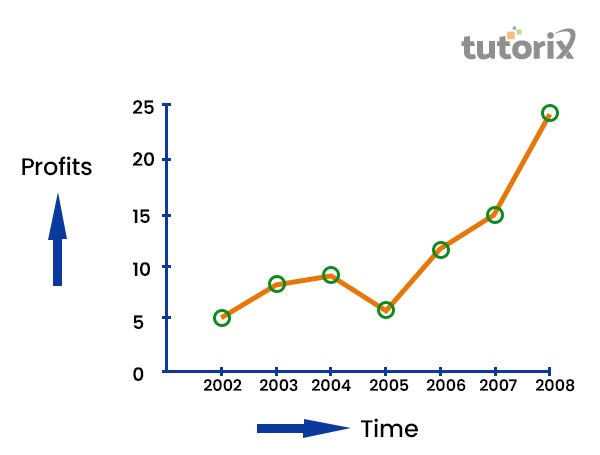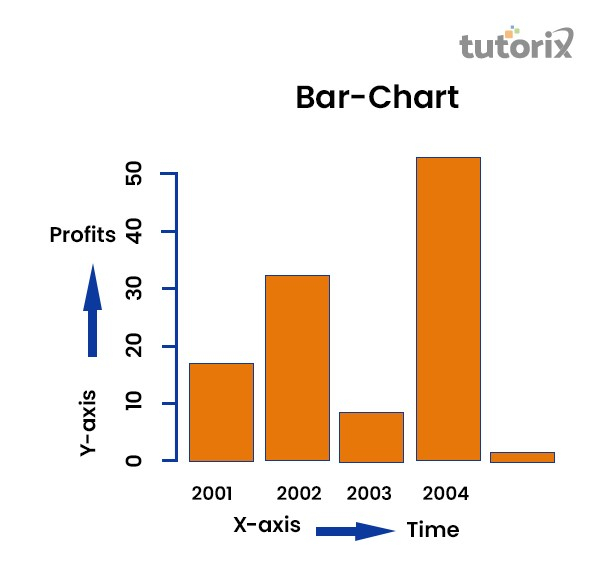# Diagrammatic Presentation Of Data

## Introduction

The diagrammatic representation also helps in having a bird’s eye view or overall view of the differentiation of data. It is a norm to present statistical data in the form of diagrams so that it becomes easier to comprehend and understand them. Therefore, diagrammatic representation is an important tool in statistics.

## What is a Diagrammatic Presentation of Data?

Diagrammatic representation refers to a representation of statistical data in the form of diagrams. The diagrams used in representing statistical data are geometrical figures, such as lines, bars, and circles. The intention of using geometrical figures in statistical presentation is to make the study more interesting and easy to understand. Diagrammatic representations are widely used in statistics, economics, and many other fields of study.

## Types of Diagrammatic Presentations of Data

Various types of diagrammatic representations of data depend on the dataset and the particular statistical elements in them. Data presentation can be made in different types and forms.

These can be broadly classified into the following one-dimensional types −

### Line Diagram

In a line diagram, straight lines are used to indicate various parameters. Here, a line represents the sequence of data associated with the changing of a particular variable.

Properties of Line Diagram −

• The Lines are either in vertical or horizontal directions.

• There may be uniform scaling but this is not mandatory.

• The lines that connect the data points offer the statistical representation of data.

The following is an example of a line diagram that shows profits in Rs crore from 2002 till 2008. Profit in 2002 was Rs 5 Crore while in 2008 it was Rs 24 Crore.### Bar Diagram

Bar diagrams have rectangular shapes of equal width that represent statistical data in a straightforward manner. Bar diagrams are one of the most widely used diagrammatic representations.

Properties of Bar Diagram −

• The Bars can be vertical or horizontal in directions.

• All bars in a diagram have a uniform width.

• All the Bars have a common and same base.

• The height or width of the Bar shows the required value.

The following is an example of a Bar Chart that has time on the X axis and profits on the Y axis.### Pie Chart

Also known as a "circle chart", the pie chart divides the circular statistical graphic into sectors or sections to illustrate the numerical data. Each sector in the circle denotes a proportionate part of the whole. Pie-chart works the best at the time when we want to denote the composition of something. In most cases, the pie chart replaces other diagrammatic representations, such as the bar graph, line plots, histograms, etc.

In practice, the various sections in a pie chart are derived according to their ratio to the total area of the circle. Then according to their individual contributions, sections are divided into parts derived from 360 degrees of the circle.

## Advantages of Diagrammatic Presentation of Data

### Easier to understand

Pictorial representations are usually easier to understand than statistical text or representation in tabular form. One can easily understand which portion or part has more contribution toward the overall dataset. This helps in understanding the data better.

The creators of diagrams usually keep the simplicity of presentation in mind to offer more information to readers. That is why diagrams are easier to comprehend than texts and tables.

### More attractive

Pictorial or diagrammatic representations of datasets are more attractive than normal representations. As colors and various other tools can be incorporated into diagrams, they become more attractive and comprehensible for the readers.

Moreover, as diagrams can be made more interactive with the help of computer graphics, they have become more acceptable and attractive currently.

### Simpler presentations

Data can be presented more simply in diagrammatic form. Both extensive unstable data and smaller complex data can be represented by diagrammatic representations more easily. This helps statisticians offer more value to their findings.

### Comparison is easier

When two or more data are compared, it is easier to do so in pictorial form. As diagrams clearly show the portion of data consumed, it can be easily understood from the diagrams which part of the data is consuming more area in the diagrams. This can help one to understand the real differences through pictorial comparison.

### Universal acceptance

Diagrammatic representation of data is used in many fields of study, such as statistics, science, commerce, economics, etc. So, the diagrams are accepted universally and hence are used everywhere.

Moreover, since there are the same procedures for forming diagrams, the representations mean the same thing to everyone. So, there is nothing to alter when we obtain the diagrams to check the real values. It helps analysts solve problems universally.

### Improvement in presentation

Diagrammatic representations improve the overall representation of data to a large extent. As the data is classified into several groups and presented in a systematic manner in diagrams, the whole presentation of data gets improved during the diagrammatic representation.

Moreover, as diagrams can be made more interactive than texts or tables, diagrammatic presentations are one step ahead in presenting the data in a simpler yet recognizable manner.

### More organized and classified data

To represent data in diagrams, they must be organized and classified into comprehensive categories. This helps the data to be organized in a given fashion which makes them orderly and creates a sequence. This in turn helps realize diagrammatic data better than text forms.

## Relevance Diagrammatic Presentation of Data

• Diagrams are a great way of representing data because they are visually attractive and they can make large, complex datasets look simpler. The otherwise heavy data can be simply and easily represented by line and bar diagrams, and pie charts. This makes data organization simpler and neater.

• Moreover, as data must be classified before representation, one must organize them according to the norms required. So, diagrammatic representations save lots of time and resources.

• Diagrams also have universal acceptance and so can be used to express data in different forms. This provides the analysts and researchers flexibility to present data in any required form.

• Diagrams also remove confusion and offer a simpler tactic to present data. As no special skill has to be learned to represent data in diagrams, they can be used by most to show statistical data and results of various types of research and experiments.

• Therefore, diagrammatic representation has great relevance that can be used for the benefit of economists, statisticians, marketing analysts, and a lot of other professionals.

## Conclusion

The diagrams are a central part of statistics and their importance can be known from the fact that almost all statistical researchers use them in one way or the other. The diagrammatical representations make inferring statistical data much simpler and easier. It is a much easier way to visualize and understand data in simpler forms too.

To represent data in diagrammatic form, only a simple understanding of Mathematics is required. So, no special skills are needed to use diagrams and this makes them very popular tools for the representation of data sets. Learning how to present data in diagrams, therefore, should be a priority for everyone.

## FAQs

Q1. Which is the simplest diagrammatic presentation of data?

Ans. The simplest diagrammatic presentation of data is a line diagram that shows data in terms of straight lines.

Q2. What are the two characteristics of bar diagrams?

Ans. Bar diagrams have uniform width and their base remains the same.

Q3. How are the sections in a pie chart formed?

Ans. In practice, the various sections in a pie chart are derived according to their ratio to the total area of the circle. Then according to their individual contributions, sections are divided into parts derived from 360 degrees of the circle.

For example, if a section requires 25% of the presentation, it will consume  degrees on the chart.

Updated on: 13-Dec-2022

7K+ Views